# Sentiment Classifier using Tfidf

This article is 2nd in the series Everything to get started with NLP. Since I have explained all the theory in part one, I will not be explaining again here.

Note: I am assuming that you have some experience with Python coding.

In this article, we will be making a Sentiment classifier. So without further adieu let us dive in.

# The Data set

`!wget http://ai.stanford.edu/~amaas/data/sentiment/aclImdb_v1.tar.gz`

You will get something like this:

The downloaded dataset is in a tar file. Let’s extract it using the shutil module. The `shutil` module offers a number of high-level operations on files and collections of files. In particular, functions are provided which support file copying and removal. For more info click here

`import shutilshutil.unpack_archive("aclImdb_v1.tar.gz", "/content/")`

If you did everything right, you will have a file structure like this:

## Overview of the dataset

• This is the IMDB movie review dataset. This dataset is annotated with positive and negative labels thanks to researchers at Stanford. The dataset can be accessed at this link.
• This dataset contains 25000 positive and 25000 negative examples that are pre annotated with labels. This dataset also contains un-annotated examples which can be used by researchers for further use.
• In both train and test folders, we will have 12,500 positives and 12,500 negative examples. Please note that each example is a movie review and it is in a separate text file. So we will have 25K text files for training and testing each.
• Dataset contains at most 30 reviews per movie. To make sure no single movie becomes more influential.
• If the movie is given more than 7 stars then it is a positive review, and if lower than 4 then negative reviews.
• Positive and negative examples are equal in number, so accuracy can be used as metrics.

We will download wordnet and stopwords packages. Both these packages will be necessary for text preprocessing. These are provided by NLTK.

`import nltknltk.download('wordnet')nltk.download('stopwords')`

Let us import other important stuff.

`import osimport pandas as pdimport numpy as npfrom nltk.corpus import stopwordsfrom sklearn.feature_extraction.text import TfidfVectorizerfrom sklearn.linear_model import LogisticRegression`

# DataFrames from Dataset

Let us make Training and Testing DataFrames from the given dataset. Here we will read files from the folder and mark them with corresponding labels in the DataFrame. For example, in the pos folder inside Train folder, we have 12,500 reviews. All of them are positive reviews. We will make a Pandas DataFrame which will have two columns:
1. Reviews: The text of the file
2. labels: 1
Since all the reviews are positive, we will have 12,500 rows in labels column as 1.

`path = "/content/aclImdb/train/pos/"temp = []for file in os.listdir(path):    with open(os.path.join(path + file), "r") as f:        temp.append(f.readlines())pos = pd.DataFrame({"reviews": temp,                "labels": list(np.ones(len(first_1000), dtype=int))})`

Things will get clear with the below image. As explained, we have movie reviews in the reviews column and 1ns in the labels column.

Now, similarly create a DataFrame for negative reviews of training data or you can directly merge in this dataset. Whatever you do at the end you should have a dataset train_data (you can choose any name of your choice) where first half rows have positive reviews and second half have negative reviews. Though you can jumble the rows also. Whatever you like.

At the end you should have labels distribution Something like this:

As you can see, we have an equal number of positive and negative examples. Similarly, create a dataset test_data for testing.

# Preprocessing

We will make some helper functions for the following preprocessing tasks:

Tokenization

`def tokenize_data(dataset):    tokenizer = nltk.tokenize.TreebankWordTokenizer()    for i in range(dataset.shape):       dataset["reviews"][i] = tokenizer.tokenize(dataset["reviews"][i])    return dataset`

Stop words removal

`def remove_stop_words(dataset):    stop_words = set(stopwords.words('english'))    for i in range(dataset.shape):        dataset["reviews"][i] = ([token.lower() for token in dataset["reviews"][i] if token not in stop_words])        return dataset`

Normalization

`def normalize(dataset):    lemmatizer = nltk.stem.WordNetLemmatizer()    for i in range(dataset.shape):        dataset.reviews[i] = " ".join([lemmatizer.lemmatize(token) for token in dataset.reviews[i]]).strip()    return dataset`

Punctuation and Symbols removal

`def remove_garbage(dataset):    garbage = "~`!@#\$%^&*()_-+={[}]|\:;'<,>.?/"    for i in range(dataset.shape):    dataset.reviews[i] = "".join([char for char in dataset.reviews[i] if char not in garbage])    return dataset`

Please Note: This creating separate functions for similar processing is pretty bad coding practice. This is just for clear explanation. We can do all this in very small number of steps. And surely within a single function with very less computational complexity.

After creating those functions(you can do in a single function), apply those functions on your datasets.

`train_data = tokenize_data(train_data)train_data = remove_stop_words(train_data)train_data = normalize(train_data)train_data = remove_garbage(train_data)test_data = tokenize_data(test_data)test_data = remove_stop_words(test_data)test_data = normalize(test_data)test_data = remove_garbage(test_data)`

The state of your dataset should be something like this.

This dataset is still not completely processed. Since this article is not for text processing and even this much processing will yield good results will leave further processing for any other day.

# Feature Extraction

Let us now extract features. We will be using TfidfVectorizer module from sklearn library. We can create our own tfidf function, but it will be a futile effort in creating something which is already present and works very well.

The first step is fitting the vectorizer. We will fit the vectorizer on the complete dataset. That is on all the reviews(train and test). We can fit the vectorizer on just Training dataset but that can have negative implications. As some words which were not present in Training can be there in Test Dataset. This function will do that for us.

`def fit_corpus(train_data, test_data):    corpus = pd.DataFrame({"reviews": train_data["reviews"]})    corpus.reviews.append(test_data["reviews"], ignore_index=True)    tfidf = TfidfVectorizer(min_df=2, max_df=0.5, ngram_range=(1,2))    tfidf.fit(corpus["reviews"])    return tfidf`

Followed by Fitting, we will transform our dataset. This function transforms our dataset into tfidf vectors.

`def transform_data(tfidf, dataset):    features = tfidf.transform(dataset["reviews"])    return pd.DataFrame(features.todense(), columns = tfidf.get_feature_names())`

Now let us call the above two functions and transform our datasets. Also, we will take training and testing labels in separate variables.

`tfidf = fit_corpus(train_data, test_data)  #Fitting the vecorizertrain_features = transform_data(tfidf, train_data)  #transforming test_features = transform_data(tfidf, test_data)    #Train and Testtrain_labels = train_data["labels"]  #Taking lables in separatetest_labels = test_data["labels"]    #variables`

The dataset should look something like this:

# Training the Model

Since we have converted text into features, we can apply any classification model on our train Dataset. Since the data is in long sparse matrix, simple logistic Regression works well.

First, we will initialize the model:

`clf = LogisticRegression(random_state=0, solver='lbfgs')`

You can explore more about other parameters of LogisticRegression from the docs.

The next step is fitting the model on training data.

`clf.fit(train_features, train_labels)`

Looks like we are done. We have made the Sentiment classification model.

Let us give some input and check the outputs:

Let’s check what is the accuracy on the test DataSet:

We got a score of 84.25 %. Eureka !!!

Without much of pre-processing and with one of the basic models, we got great results. ML is so easy!!!

If you are thinking this then:

ML is much more than the score. By running a model using some pre-built library is not ML. Explore more, learn more.

I said that because we got that accuracy as the dataset was already in pretty good shape. And If you really wanna challenge yourself then try taking the score up.

You can tune the parameters or use different algorithms or can clean the dataset more. Whatever you prefer.

Just don’t OVERFIT .

## Some Notes on improving the results of Logistic Regression

1. Play around with tokenization: Special tokens for emojis, exclamations, etc. can help. These are some tokens which people often use and can tell a lot about the sentiments in a review. For example, a smiling emoji can be a very good indicator of a good review, whereas sad or angry emoji could point towards a bad review.
2. Try different models like SVM, Naive Bayes, etc.
3. Throw BOW away and use Deep Learning. Though accuracy gain from Deep learning for this sentiment analysis task is not mind-blowing.

# Some Notes on implementation

1. This tfidf vectorization and text pre-processing take a good amount of processing. Try to use Google Colab. It’s free and gives GPU and TPU support with 25GB of RAM.
2. But if you have other resources then you can always use them.
3. You can get the code on my Github.

If you liked this article then you can hit that CLAP button as many times as you like. Also, you can connect with me on LinkedIn or follow me on GitHub.

--

--

--

## More from Analytics Vidhya

Analytics Vidhya is a community of Analytics and Data Science professionals. We are building the next-gen data science ecosystem https://www.analyticsvidhya.com

## Deep Hedging and GANs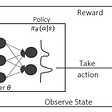## Let the machine price your car.## Day 43: 60 days of Data Science and Machine Learning Series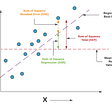## Report — examples & model answers | B2 First (FCE)## Accelerate your Deep Learning Pipeline with NVIDIA Toolkit## Unlock the Power of XGBoost## Read with me: Point Transformer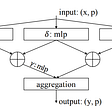## A Look at Linear Regression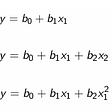## Sentiment Analysis with CNN using keras## END TO END MODEL(ML & DL) BUILDING FOR TWEET CLASSIFICATION AND DEPLOYMENT USING STREAMLIT## Text Preprocessing in NLP With Python — II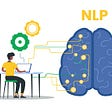## Untitled